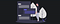# An Introduction to Dynamic ProgrammingThose who cannot remember the past are condemned to repeat it-Dynamic Programming

Although people make a big deal about how scary dynamic programming problems are, there’s really no need to be afraid of them. In fact, once you get the hang of them, these can actually be very easy problems.

What is dynamic programming?

Dynamic programming is a technique to solve problems by breaking it down into a collection of sub-problems, solving each of those sub-problems just once and storing these solutions inside the cache memory in case the same problem occurs the next time. Dynamic Programming is mainly an optimization over plain recursion . Wherever we see a recursive solution that has repeated calls for same inputs, we can optimize it using Dynamic Programming. This simple optimization reduces the time complexities from exponential to polynomial.

Briefly speaking, dynamic programming is mostly just a matter of taking a recursive algorithm and finding the overlapping subproblems (that is, the repeated calls). You then cache those results for future recursive calls. Alternatively, you can study the pattern of the recursive calls and implement something iterative. You still “cache” previous work.

There are two different ways to store our values so that they can be reused. Let’s discuss both of them:
1. Tabulation or the Bottom Up approach.
2. Memoization or the Top Down Approach.

A note on terminology: Some people call top-down dynamic programming “memoization” and only use “dynamic programming” to refer to bottom-up work.

Before getting to the definitions of the above two terms consider the below statements:

• Version 1: I will study the theory of Dynamic Programming , then I will practice some problems and hence I will master Dynamic Programming.

Both these versions essentially convey the same meaning but the difference lies in the way they are approached.

Let’s begin with a simple problem statement to understand these more clearly.
The Fibonacci numbers are the numbers in the following integer sequence.

0, 1, 1, 2, 3, 5, 8, 13, 21, 34, 55, 89, 144, ……..

In mathematical terms, the sequence Fn of Fibonacci numbers is defined by the recurrence relation

Fn = Fn-1 + Fn-2

with seed values

F0 = 0 and F1 = 1.

## If we code the solution to this without using dp:

It can be observed that we are repeating numerous function calls, thereby increasing our time complexities to O(2^n). Actually, it’s slightly better than O(2^n) .If you look at the subtree, you might notice that (excluding the leaf nodes and those immediately above it) the left subtree of any node is always smaller than the right subtree. If they were the same size, we’d have an O( 2^n) runtime. But since the right and left subtrees are not the same size, the true runtime is closer to O( 1. 6^n). Saying O( 2^n) is still technically correct though as it describes an upper bound on the runtime. But still this is a major issue.

Tabulation :-
In the first version, we start our journey from the building blocks and continue by cumulating our answers all the way to the top.

Coding the solution to the above problem statement using Tabulation(bottom up):

Memoization:-
In the second version, we start our journey from the top most destination state and compute its answer by taking in count the values of states that can reach the destination state, till we reach the bottom most base state.

Coding the solution to the above problem statement using Memoization(top down):

Now , let’s take a look at how our runtime was improved using dp.

It can be clearly observed that by caching our values into the array, we reduced a lot of function calls thereby reducing our time complexity from exponential to linear. Our runtime was reduced to O(n). This is actually a great achievement.

How to check if a program can use Dynamic Programming?

Now, that we know how to solve problems using dp, let’s discuss briefly about when to use this technique. If we are reading the problem and come across the following questions , I believe there is a way to implement dp in there.
1. Can the problem be divided into sub-problems?
2. Is there a recursive solution to the problem?
3. Are there repetitive sub-problems?
If the answers to all of the above questions is a YES, you are good to go.

## Practice problems:

Now that you know what dp is and how to solve a problem using the technique, why don’t you try out these questions:
1.https://leetcode.com/problems/house-robber/
2.https://leetcode.com/problems/climbing-stairs

# Conclusion

Most of the problems you’ll encounter within Dynamic Programming already exist in one shape or another. Often, your problem will build on from the answers for previous problems. Here’s a list of common problems that use Dynamic Programming.

I hope that whenever you encounter a problem, you think to yourself “can this problem be solved with ?” and try it.

# Resources

There are a whole host of resources out there on Dynamic Programming, if you just know what to Google for when you search for them. If you’re looking for some further reading , the links below are a good place to start.

As always,in addition to these, the bibles of CP namely Cracking the Coding Interview by Gayle Laakmann McDowell and Programming Interviews Exposed by John Mongan, Noah Suojanen and Eric Giguere are a must.

Written by

Written by

## Jay Prakash

#### Student, College of Engineering and Technology, Bhubaneswar